# Rectangular garden

The perimeter of Peter's rectangular garden is 98 meters. The width of the garden is 60% shorter than its length.
Find the dimensions of the rectangular garden in meters.
Find the garden area in square meters.

Result

a =  35 m
b =  14 m
S =  490 m2

#### Solution:

$o = 98 \ m \ \\ b = a - 0.60a \ \\ \ \\ o = 2(a+b) \ \\ \ \\ \ \\ o = 2 \cdot \ (a+(a - 0.60 \cdot \ a)) \ \\ \ \\ 98 = 2 \cdot \ (a+(a - 0.60 \cdot \ a)) \ \\ \ \\ 2.8a = 98 \ \\ \ \\ a = 35 \ \\ = 35 \ \text{ m }$
$b = a - 0.60a = 35 - 0.60 \cdot \ 35 = 14 = 14 \ \text{ m }$
$S = a \cdot \ b = 35 \cdot \ 14 = 490 = 490 \ m^2$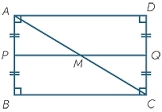Our examples were largely sent or created by pupils and students themselves. Therefore, we would be pleased if you could send us any errors you found, spelling mistakes, or rephasing the example. Thank you!

Leave us a comment of this math problem and its solution (i.e. if it is still somewhat unclear...):Be the first to comment!Tips to related online calculators
Do you have a linear equation or system of equations and looking for its solution? Or do you have quadratic equation?

## Next similar math problems:

1. Two patches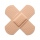Peter taped the wound with two rectangular patches (one over the other to form the letter X). The area sealed with both patches at the same time had a content of 40cm2 and a circumference of 30cm. One of the patches was 8cm wide. What was the width of tThe quadrilateral pyramid has a rectangular base of 24 cm x 3.2dm and a body height of 0.4m. Calculate its volume and surface area.
3. Cross five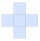The figure on the picture is composed of the same squares and has a content of 45cm². What's his perimeter?
4. The schoolyardThe schoolyard had the shape of a square with an 11m side. The yard has been enlarged by 75 m2 and has a square shape again. How many meters was each side of the yard enlarged?
5. Two bodiesThe rectangle with dimensions 8 cm and 4 cm is rotated 360º first around the longer side to form the first body. Then, we similarly rotate the rectangle around the shorter side b to form a second body. Determine the ratio of surfaces of the first and seco
6. Squares above sidesTwo squares are constructed on two sides of the ABC triangle. The square area above the BC side is 25 cm2. The height vc to the side AB is 3 cm long. The heel P of height vc divides the AB side in a 2: 1 ratio. The AC side is longer than the BC side. Calc
7. Diagonal intersectisosceles trapezoid ABCD with length bases | AB | = 6 cm, CD | = 4 cm is divided into 4 triangles by the diagonals intersecting at point S. How much of the area of the trapezoid are ABS and CDS triangles?
8. Two 2D shapes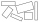Decide which shapes have more area:   (a) a square of 8cm side; or   (b) two rectangles with sides 5cm and 15cm? Write result as 1 or 2 (rectangles)
9. Octagonal pyramidFind the volume of a regular octagonal pyramid with height v = 100 and the angle of the side edge with the plane of the base is α = 60°.
10. Tetrahedral pyramidDetermine the surface of a regular tetrahedral pyramid when its volume is V = 120 and the angle of the sidewall with the base plane is α = 42° 30´.
11. Uboid volumeCalculate the cuboid volume if the walls are 30cm², 35cm², 42cm²
12. Eq triangle minus arcsIn an equilateral triangle with a 2cm side, the arcs of three circles are drawn from the centers at the vertices and radii 1cm. Calculate the content of the shaded part - a formation that makes up the difference between the triangle area and circular cuts
13. Mysterious areaThe trapezoid ABCD is given. Calculate its area if the area of the DBC triangle is 27 cm2.
14. Hexagon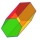Calculate the surface of a regular hexagonal prism whose base edge a = 12cm and side edge b = 3 dm.
15. The parabolic segment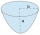The parabolic segment has a base a = 4 cm and a height v = 6 cm. Calculate the volume of the body that results from the rotation of this segment a) around its base b) around its axis.
16. Paint cansHow many paint cans do we need to paint the floor in two rooms with dimensions of 6.8m x 4.5m and 6m x3.8m? One can arefor 6m².
17. RectanglesHow many different rectangles can be made from 60 square tiles of 1 m square? Find the dimensions of these rectangles.